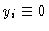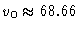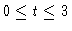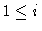## Railway Bogie

Original source of the model: N.K. Cooperrider: The Hunting Behavior of Conventional Railway Trucks. Transactions of the ASME; Journal of Engineering for Industry 94 (1972), 752-762

Further references: Ch. Kaas-Petersen: Chaos in a Railway Bogie. ACTA Mechanica 61 (1986), 89-107 U. Galvanetto, L. Briseghella, S.R. Bishop: Optimal Axle Distance of a Railway Bogie. Int. J. Bifurcation & Chaos 7 (1997), 721-732

The number of freedoms of this wheelset is 7, and the system consists of 14 first-order differential equations. The stationary solutionfor all i loses its stability for the parameter valuemph through a Hopf bifurcation with a hard loss of stability. For a special initial state the transition to the stable periodic orbit is illustrated in Figure 2, whereas in Figure 3 the oscillation is attracted by the stable stationary state. As has been pointed out by Brian Hassard, the Hopf bifurcation is tricky as there are problems with smoothness at the origin. This is explained in Hassard's worksheet, where also the equations and other information is found.

Figure 1
A railway bogie with two axles, schematically

Figure 2
attracting periodic orbit for v=70, y5(t) for, initial vector y1(0)=0.1, yi(0)=0 forFigure 3
transient phase close to the attracting stationary state for v=60, y5(t) for, initial vector y1(0)=0.1, yi(0)=0 forThis document
Figure 1
Figure 2
Figure 3